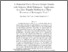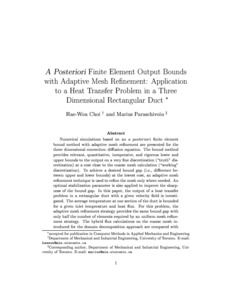Title:

# A posteriori finite element output bounds with adaptive mesh refinement: application to a heat transfer problem in a three-dimensional rectangular duct

Choi, Hae-Won and Paraschivoiu, Marius (2002) A posteriori finite element output bounds with adaptive mesh refinement: application to a heat transfer problem in a three-dimensional rectangular duct. Computer Methods in Applied Mechanics and Engineering, 191 (43). pp. 4905-4925. ISSN 0045-7825Preview
Text (post-print) (application/pdf)
duct.pdf - Submitted Version
819kB

## Abstract

Numerical simulations based on an a posteriori finite element bound method with adaptive mesh refinement are presented for the three-dimensional convection–diffusion equation. The bound method provides relevant, quantitative, inexpensive, and rigorous lower and upper bounds to the output on a very fine discretization (“truth” discretization) at a cost close to the coarse mesh calculation (“working” discretization). To achieve a desired bound gap (i.e., difference between upper and lower bounds) at the lowest cost, an adaptive mesh refinement technique is used to refine the mesh only where needed. An optimal stabilization parameter is also applied to improve the sharpness of the bound gap. In this paper, the output of a heat transfer problem in a rectangular duct with a given velocity field is investigated. The average temperature at one section of the duct is bounded for a given inlet temperature and heat flux. For this problem, the adaptive mesh refinement strategy provides the same bound gap with only half the number of elements required by an uniform mesh refinement strategy. The hybrid flux calculation on the coarse mesh introduced for the domain decomposition approach is compared with the hybrid flux calculation on the fine mesh to analyze the contribution of the hybrid flux to the bound gap.

Divisions: Concordia University > Gina Cody School of Engineering and Computer Science > Mechanical and Industrial Engineering Article Yes Choi, Hae-Won and Paraschivoiu, Marius Computer Methods in Applied Mechanics and Engineering September 2002 10.1016/S0045-7825(02)00420-6 6750 ANDREA MURRAY 02 Jul 2010 15:18 18 Jan 2018 17:29

## References:

1. M. Ainsworth and J.T. Oden , A unified approach to a posteriori error estimation using element residual methods. Numer. Math. 65 (1993), pp. 23–50.

2. H.-W. Choi, A posteriori finite element bounds with adaptive mesh refinement: application to outputs of the three dimensional convection–diffusion equation, Master’s thesis, University of Toronto, 2001.

3. C. Farhat and F.-X. Roux , A method of finite tearing and interconnecting and its parallel solution algorithm. Int. J. Numer. Methods Engrg. 32 (1991), pp. 1205–1227.

4. C. Farhat and F.-X. Roux , Implicit parallel processing in structural mechanics. Comput. Mech. Adv. 2 1 (1994), pp. 1–124.

5. P. Ladevèze and D. Leguillon , Error estimation procedures in the finite element method and applications. SIAM J. Numer. Anal. 20 (1983), pp. 485–509.

6. L. Machiels, A.T. Patera and J. Peraire , A posteriori finite element output bounds for the incompressible Navier–Stokes equations; application to a natural convection problem. J. Comput. Phys. 172 (2001), pp. 401–425.

7. Y. Maday, A.T. Patera and J. Peraire , A general formulation for a posteriori bounds for output functionals of partial differential equations; application to the eigenvalue problem. C. R. Acad. Sci.-Math 328 (1999), pp. 823–828.

8. M. Paraschivoiu, A posteriori finite element bounds for linear-functional outputs of Coericive Partial Differential Equations and of the Stokes Problems, Ph.D. thesis, Department of Mechanical Engineering, Massachusetts Institute of Technology, 1997.

9. M. Paraschivoiu , A posteriori finite element output bounds in three space dimensions using the FETI method. Comput. Methods Appl. Mech. Engrg. 190 (2001), pp. 6629–6640.

10. M. Paraschivoiu and A.T. Patera , A hierarchical duality approach to bounds for the outputs of Partial Differential Equations. Comput. Methods Appl. Mech. Engrg. 158 (1998), pp. 389–407.

11. M. Paraschivoiu and A.T. Patera , A posteriori finite element bounds for linear-functional outputs of the Stokes Problem. Int. J. Numer. Methods Fluids 32 (2000), pp. 823–849.

12. M. Paraschivoiu, A.T. Patera and J. Peraire , A posteriori finite element bounds for linear-functional outputs of elliptic Partial Differential Equations. Comput. Methods Appl. Mech. Engrg. 150 (1997), pp. 289–312.

13. M. Paraschivoiu, A.T. Patera, Y. Maday and J. Peraire , Fast bounds for Partial Differential Equation outputs. In: J. Borggaard, J.A. Burns, E.M. Cliff and S. Schreck, Editors, Computational Methods for Optimal Design and Control, Birkhäuser (1998), pp. 323–360.

14. A.T. Patera, J. Peraire. Bounds for linear-functional outputs of Coercive Partial Differential equation: local indicators and adaptive refinement, in: P. Ladeveze, J.T. Oden (Eds.), Proceedings of the Workshop On New Advances in Adaptive Computational Methods in Mechanics, Cachan, 17–19 September, 1997, Elsevier.

15. A.T. Patera and J. Peraire , Asymptotic a posteriori finite element bounds for the outputs of noncoercive problems: the Helmholtz and Burgers equation. Comput. Methods Appl. Mech. Engrg. 171 (1999), pp. 77–86.

16. N.A. Pierce and M.B. Giles , Adjoint recovery of superconvergent functionals from PDE approximations. SIAM Rev. 42 2 (2000), pp. 247–264.

17. R. Rannacher , Adaptive Galerkin finite element methods for partial differential equations. J. Comp. Appl. Math. 128 (2001), pp. 205–233.
All items in Spectrum are protected by copyright, with all rights reserved. The use of items is governed by Spectrum's terms of access.

Repository Staff Only: item control pageResearch related to the current document (at the CORE website)
Back to top